### Home > CAAC > Chapter 16 > Lesson 16.9.1.1 > Problem9-7

9-7.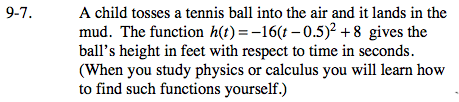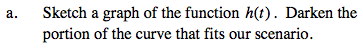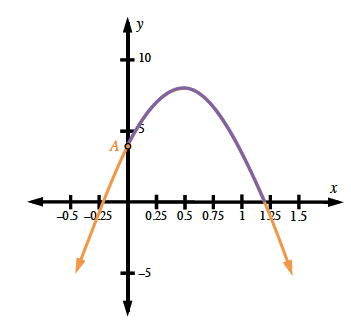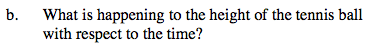First it increases, then it decreases.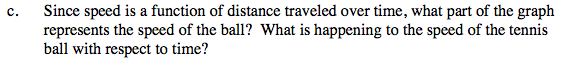The slope represents the speed of the ball. First it decreases (slope decreases). It stops momentarily (slope is at zero at the vertex) then it increases (slope gets steeper).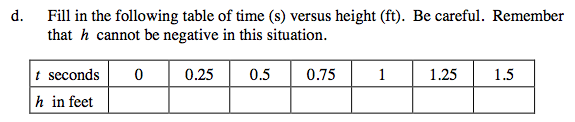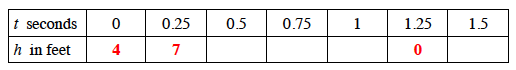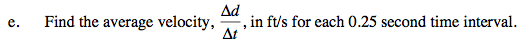Here is the first interval. Complete the other 5 intervals.

$\frac{\Delta \textit{d}}{\Delta \textit{t}} = \frac{7 - 4}{0.25 - 0} = \frac{3}{0.25} = 12$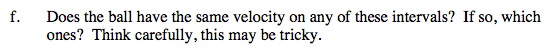No. The speeds are the same, but velocities are different (except after the ball gets stuck in the mud).